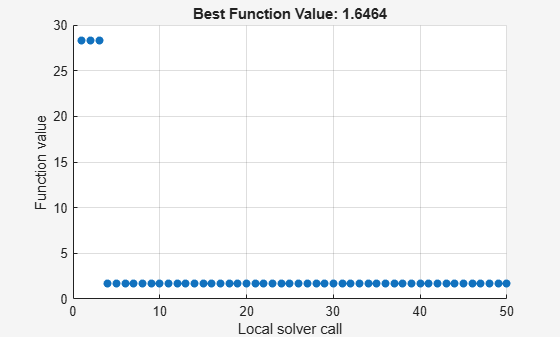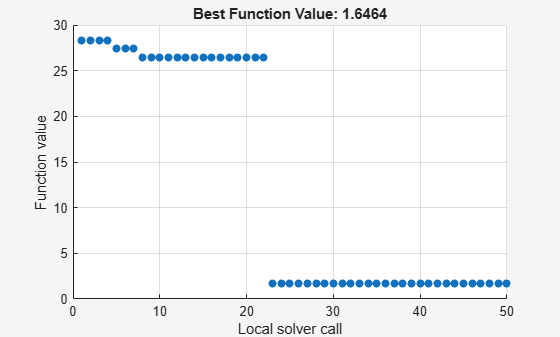Documentation

### This is machine translation

Mouseover text to see original. Click the button below to return to the English version of the page.

## MultiStart Using `lsqcurvefit` or `lsqnonlin`

This example shows how to fit a function to data using `lsqcurvefit` together with `MultiStart`. The end of the example shows the same solution using `lsqnonlin`.

Many fitting problems have multiple local solutions. `MultiStart` can help find the global solution, meaning the best fit. This example first uses `lsqcurvefit` because of its convenient syntax.

The model is

`$y=a+b{x}_{1}\mathrm{sin}\left(c{x}_{2}+d\right),$`

where the input data is $x=\left({x}_{1},{x}_{2}\right)$, and the parameters $a$, $b$, $c$, and $d$ are the unknown model coefficients.

### Step 1. Create the objective function.

Write an anonymous function that takes a data matrix `xdata` with `N` rows and two columns, and returns a response vector with `N` rows. The function also takes a coefficient matrix `p`, corresponding to the coefficient vector $\left(a,b,c,d\right)$.

`fitfcn = @(p,xdata)p(1) + p(2)*xdata(:,1).*sin(p(3)*xdata(:,2)+p(4));`

### Step 2. Create the training data.

Create 200 data points and responses. Use the values $a=-3,b=1/4,c=1/2,d=1$. Include random noise in the response.

```rng default % For reproducibility N = 200; % Number of data points preal = [-3,1/4,1/2,1]; % Real coefficients xdata = 5*rand(N,2); % Data points ydata = fitfcn(preal,xdata) + 0.1*randn(N,1); % Response data with noise```

### Step 3. Set bounds and initial point.

Set bounds for `lsqcurvefit`. There is no reason for $d$ to exceed $\pi$ in absolute value, because the sine function takes values in its full range over any interval of width $2\pi$. Assume that the coefficient $c$ must be smaller than 20 in absolute value, because allowing a high frequency can cause unstable responses or inaccurate convergence.

```lb = [-Inf,-Inf,-20,-pi]; ub = [Inf,Inf,20,pi];```

Set the initial point arbitrarily to (5,5,5,0).

```p0 = 5*ones(1,4); % Arbitrary initial point p0(4) = 0; % Ensure the initial point satisfies the bounds```

### Step 4. Find the best local fit.

Fit the parameters to the data, starting at `p0`.

`[xfitted,errorfitted] = lsqcurvefit(fitfcn,p0,xdata,ydata,lb,ub)`
```Local minimum possible. lsqcurvefit stopped because the final change in the sum of squares relative to its initial value is less than the value of the function tolerance. ```
```xfitted = 1×4 -2.6149 -0.0238 6.0191 -1.6998 ```
```errorfitted = 28.2524 ```

`lsqcurvefit` finds a local solution that is not particularly close to the model parameter values (–3,1/4,1/2,1).

### Step 5. Set up the problem for `MultiStart`.

Create a problem structure so `MultiStart` can solve the same problem.

```problem = createOptimProblem('lsqcurvefit','x0',p0,'objective',fitfcn,... 'lb',lb,'ub',ub,'xdata',xdata,'ydata',ydata);```

### Step 6. Find a global solution.

Solve the fitting problem using `MultiStart` with 50 iterations. Plot the smallest error as the number of `MultiStart` iterations.

```ms = MultiStart('PlotFcns',@gsplotbestf); [xmulti,errormulti] = run(ms,problem,50)``````MultiStart completed the runs from all start points. All 50 local solver runs converged with a positive local solver exit flag. ```
```xmulti = 1×4 -2.9852 -0.2472 -0.4968 -1.0438 ```
```errormulti = 1.6464 ```

`MultiStart` finds a global solution near the parameter values (–3,–1/4,–1/2,–1). (This is equivalent to a solution near `preal` = (–3,1/4,1/2,1), because changing the sign of all the coefficients except the first gives the same numerical values of `fitfcn`.) The norm of the residual error decreases from about 28 to about 1.6, a decrease of more than a factor of 10.

### Formulate Problem for `lsqnonlin`

For an alternative approach, use `lsqnonlin` as the fitting function. In this case, use the difference between predicted values and actual data values as the objective function.

```fitfcn2 = @(p)fitfcn(p,xdata)-ydata; [xlsqnonlin,errorlsqnonlin] = lsqnonlin(fitfcn2,p0,lb,ub)```
```Local minimum possible. lsqnonlin stopped because the final change in the sum of squares relative to its initial value is less than the value of the function tolerance. ```
```xlsqnonlin = 1×4 -2.6149 -0.0238 6.0191 -1.6998 ```
```errorlsqnonlin = 28.2524 ```

Starting from the same initial point `p0`, `lsqnonlin` finds the same relatively poor solution as `lsqcurvefit`.

Run `MultiStart` using `lsqnonlin` as the local solver.

```problem2 = createOptimProblem('lsqnonlin','x0',p0,'objective',fitfcn2,... 'lb',lb,'ub',ub'); [xmultinonlin,errormultinonlin] = run(ms,problem2,50)``````MultiStart completed the runs from all start points. All 50 local solver runs converged with a positive local solver exit flag. ```
```xmultinonlin = 1×4 -2.9852 -0.2472 -0.4968 -1.0438 ```
```errormultinonlin = 1.6464 ```

Again, `MultiStart` finds a much better solution than the local solver alone.

Watch now